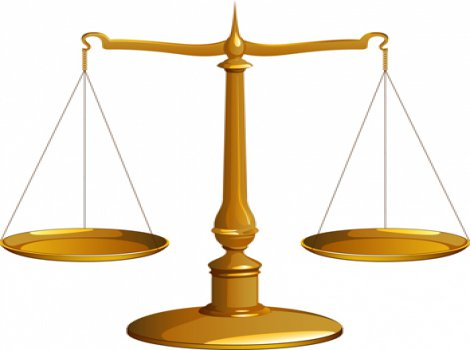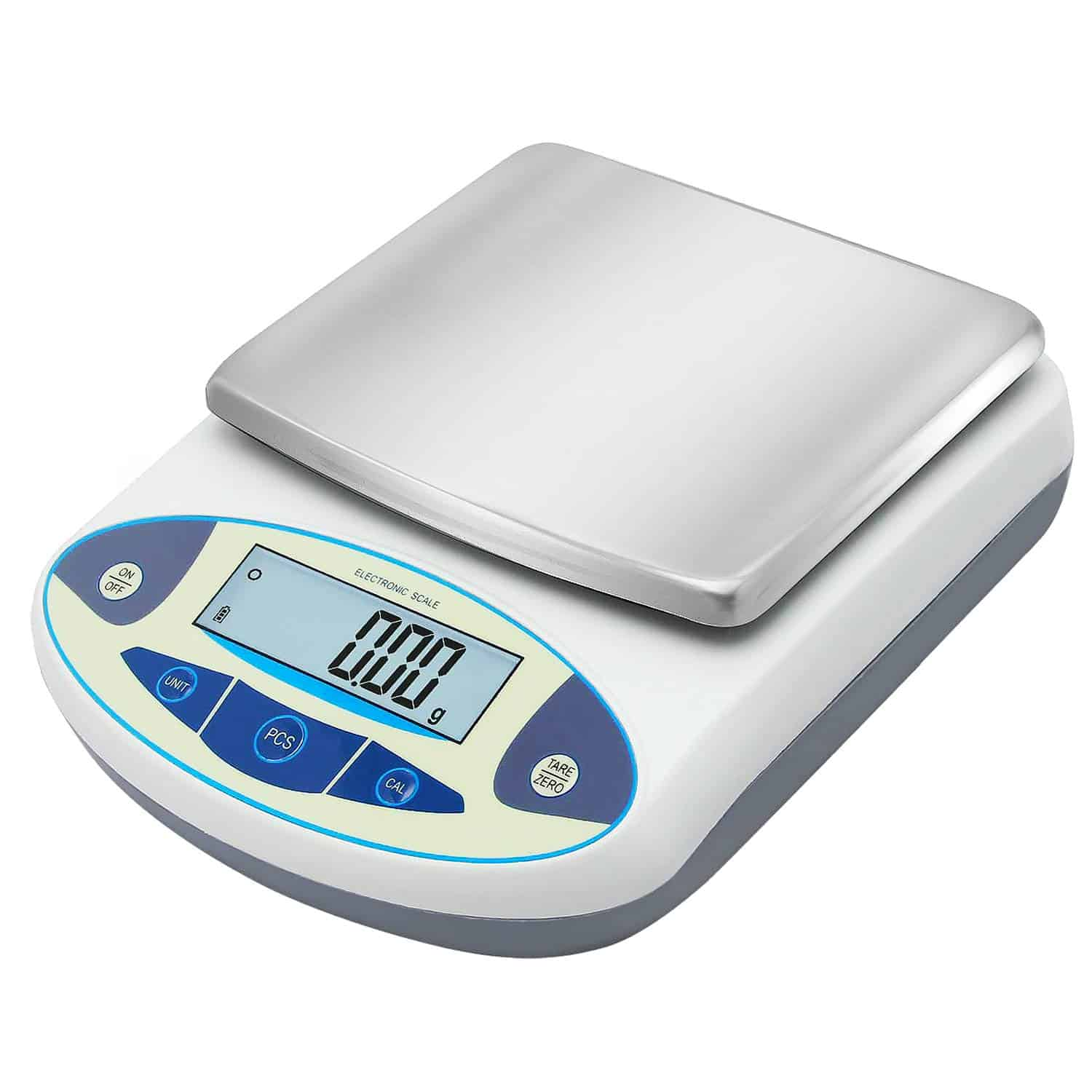# What Tool is Used to Measure Mass?The mass of an object is used to describe the amount of matter present in a body. Mass and weight are different terms in scientific discussion; the weight of a body represents the earth’s gravitational pull towards its center. To determine the mass, the scientists have devised several instruments. The use of different tools is dependent on the size, shape, or location of the object to get reliable results with little uncertainty.

## What tool is used to measure mass?

The tools for measuring the mass in different environments are:

• Balances and scales
• Vibrating tube sensors
• Newtonian mass measurement devices
• Measurement transducers## What Scientific Instrument is Used to Measure Mass?

Balance or scale is the most common tool that scientists use to determine the mass of an object. They compare the thing of a known mass with the unknown to estimate the exact mass of the object in question.

Triple balance is one example of the widely accepted balance to estimate the mass in a metric system. The standard unit for measuring the mass is a gram or kilogram based on the metric system.

The different types of balances such as scientific digital balance and a beam balance use springs to which the body of an unknown quantity is fastened. The stiffness of the spring and the level of vibration help determine the mass.

Nowadays, various modern digital and spring scales are available as home appliances. You can know your body weight just by standing on the weight balance. A digital ratio measures the body weight and gives the exact mass by subtracting the effect of gravity.## What is the Standard Unit Used to Measure Mass?

The standard unit of measuring the mass is the kilogram or gram. We represent gram as ‘g’ and kilogram as ‘kg.’ The kilogram is used to measure the mass of weighty objects like watermelon or a bottle of one-liter water, while we calculate the mass of lighter things like chocolate in grams. There are 1000 grams in one kilogram.

## Does Beam Balance Measure Mass?

Yes, the beam balance is preferably used to measure the mass of a body in the range of 10 mg to 1Kg under gravitational force for high precision. It contains a couple of scale pans at each end of a rigid beam. The pans are hung through stirrups, and a wooden baseboard is provided with three leveling screws, and two out of them are of adjustable heights that help level the baseboard horizontally.

The two masses are taken in pans to compare the weights of the body, and weight is proportional to the masses if they are determined in the same place.

## More instruments for Measuring the Mass

More instruments for objects of specific types and nature are briefly mentioned in this article.

## Space Linear Acceleration Mass Measurement Device (SLAMMD)

SLAMMD is another unworldly and less common device for measuring the mass of a human in orbit at the International Space Station. A pole-mounted device depends on Sir Isaac Newton’s Second Law of Motion, which states that force equals the mass times acceleration; the two springs apply pressure against a person to determine the person’s mass via power and acceleration.

## Measuring Transducer Device

For instance, if it is impossible to determine the mass using a balance, another substitute is available. For measuring the mass of a fluid in a graduated tank, scientists prefer using transducers. A transducer determines the characteristic mass of a drink in a steady state. It delivers a signal to the processor that proceeds the further mass calculation, and the indicator displays the mass.

The gross mass is obtained by subtracting the floating roof mass.

## Vibrating Tube Mass Sensor Tool

It is always challenging to determine the microscope properties of an object. But, modern scientifically developed tools are fruitful in dealing with the tiny, invisible, or minimal material. It is interesting to measure the micro-gram-sized biological samples in the fluid with the help of a Vibrating Tube Mass Sensor.

The first step of its functionality is the measurement of the buoyant mass of an object using the density of the fluid; once the positive group is determined, the absolute value of the mass can be found by measuring the buoyant mass of the object in liquids of various densities. This cost-effective and portable tool provides invaluable data for biomaterials such as cells, seeds, and embryos.

## Gravitational Interaction

For the gigantic objects in space, scientists believe that the gravitational interaction of the object in question with nearby objects is reliable in determining the mass. To estimate the mass of a star, you need to know the distance plus time of its movement concerning others. Scientists also use the velocity of rotation to calculate the mass of different galaxies.

## Which of these is an Appropriate Instrument to Measure Mass?

The balances and scales are widely used reliable tools for measuring the exact mass of an object with little uncertainty. They are easy to use for all kinds of things, less costly, and more reliable than any other complex instrument.

Suppose you use beam balance place in the left pan the object whose mass is determined with standard known mass in the right pan. Adjust them so that the beam is horizontal while holding the balance up. The standard net weight is equal to the mass of the given body.

As we see, it measures the mass of an object by just comparing it with a known group, and there is no effect of gravity or experimental error.

The digital balance has taken over the massive sphere of the scientific world due to its convenience, quickness, and reliability. If you are endeavoring to gain or lose weight and check the variations periodically, you can determine your body weight in numbers by placing yourself on the digital scale.

The digital balance helps scientists calculate the exact amount of chemicals required to use for a particular experiment in the laboratory.

Inflation Is Eating IRA/401(k) Savings! How to Protect Your IRA/401(k) in Bad Times?